Question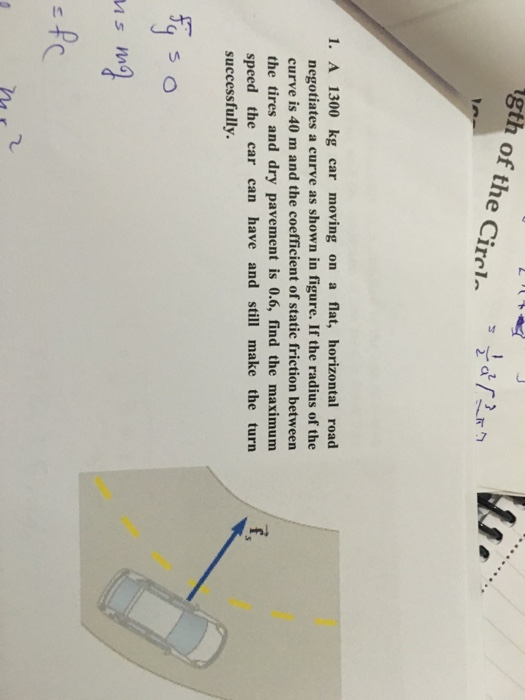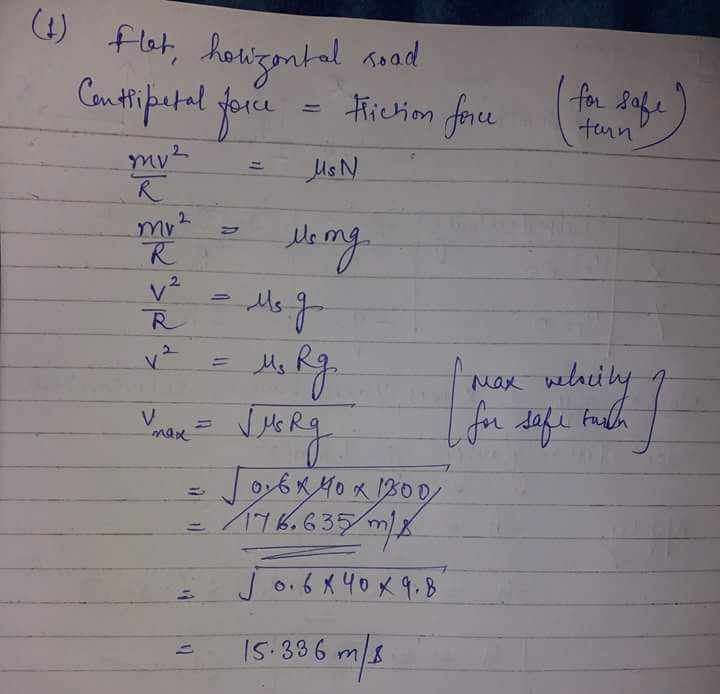#### Earn Coins

Coins can be redeemed for fabulous gifts.

Similar Homework Help Questions
• ### You are driving your car along a flat, curved road; the curve in the road is...

You are driving your car along a flat, curved road; the curve in the road is a segment of a circle with radius 50 meters. (We call this a "radius of curvature"). How fast can the car drive around the curve if the coefficient of static friction between the tires and the road is 1.0 (tires on dry pavement)? What if the coefficient of friction is 0.2 (tires on ice)?

• ### 1000 kg car rounds a curve on a flat road of radius 20 m. if the...

1000 kg car rounds a curve on a flat road of radius 20 m. if the force of friction between dry pavement and tire is 5800 N, what is the maximum speed the car can safely make the turn?

• ### A car of mass M = 1300 kg traveling at 65.0 km/hour enters a banked turn...

A car of mass M = 1300 kg traveling at 65.0 km/hour enters a banked turn covered with ice. The road is banked at an angle θ, and there is no friction between the road and the car's tires as shown in (Figure 1) . Use g = 9.80 m/s2 throughout this problem. r= 91.43 m. Now, suppose that the curve is level (θ=0) and that the ice has melted, so that there is a coefficient of static friction μ...

• ### 5. A car with mass of 1200 kg rounds a flat, unbanked curve with radius of...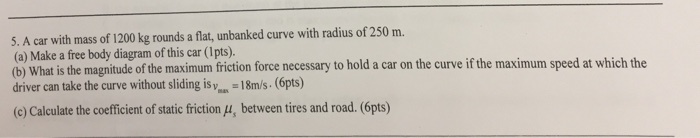5. A car with mass of 1200 kg rounds a flat, unbanked curve with radius of 250 m. (a) Make a free body diagram of this car (1pts). driver can take the curve without sliding is yos. -18m/s. (6pts) (c) Calculate the coefficient of static friction (u, between tires and road. (6pts) at is the magnitude of the maximum friction force necessary to hold a car on the curve if the maximum speed at which the

• ### PLEASE ANSWER PART B. THANKS! t Banked Frictionless Curve, and Flat Curve with Friction A car...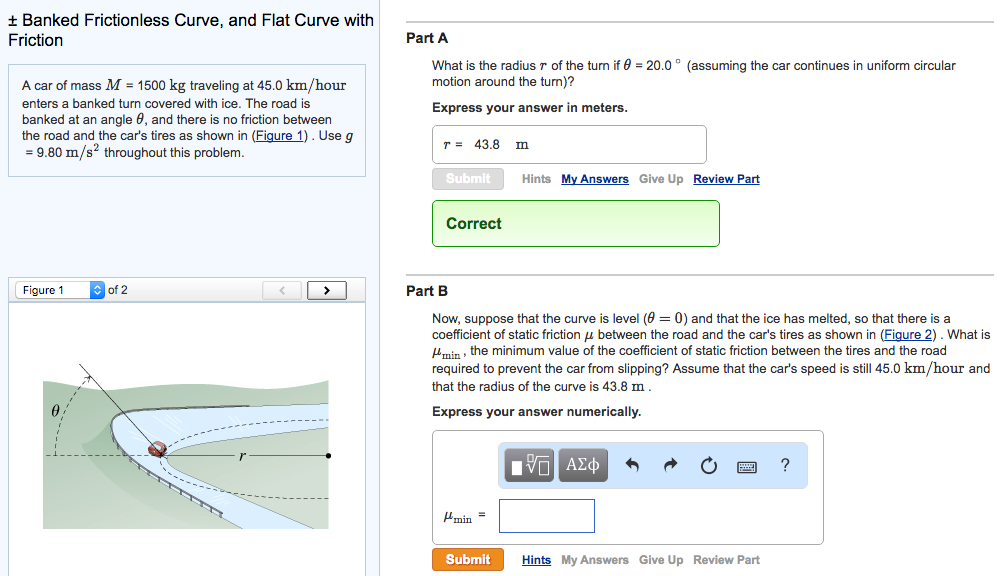PLEASE ANSWER PART B. THANKS! t Banked Frictionless Curve, and Flat Curve with Friction A car of mass M 1500 kg traveling at 45.0 km/hour enters a banked turn covered with ice. The road is banked at an angle 6, and there is no friction between the road and the car's tires as shown in (Figure 1). Use g 9.80 m/s2 throughout this problem. of 2 Figure 1 Part A What is the radius r of the turn if 0...

• ### Banked Frictionless Curve, and Flat Curve with Friction Reviev A car of mass M - 1300...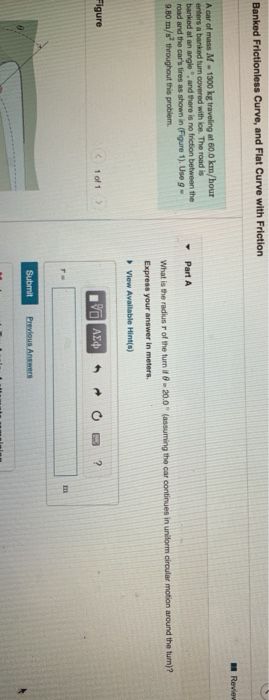Banked Frictionless Curve, and Flat Curve with Friction Reviev A car of mass M - 1300 kg traveling at 60.0 km/hour enfers a banked turn covered with ice. The road is banked at an angle and there is no friction between the road and the car's tires as shown in Figure 1). Use 9 - 9.B0 m/s throughout this problem. Part A What is the radius of the turn it 8 - 20.0 (assuming the car continues in uniform circular...

• ### What is the maximum speed with which a 1200 kg car can round a turn of...

What is the maximum speed with which a 1200 kg car can round a turn of radius 75 m on a flat road if the coefficient of static friction between tires and road is 0.80?(m/s) Is this result independent of the mass of the car?

• ### Answer all parts 2. A 1000 kg car rounds a curve of radius 50 m on...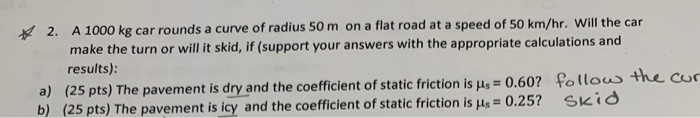Answer all parts 2. A 1000 kg car rounds a curve of radius 50 m on a flat road at a speed of 50 km/hr. Will the car make the turn or will it skid, if (support your answers with the appropriate calculations and results): a) (25 pts) The pavement is dryand the coefficient of static friction is μ,-0.60? fo too the co b) (25 pts) The pavement is icy and the coefficient of static friction is s 0.25? SKid

• ### A car of mass M = 800 kg traveling at 55.0 km/hour enters a banked turn...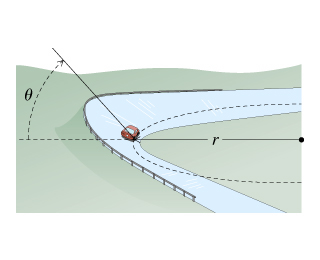A car of mass M = 800 kg traveling at 55.0 km/hour enters a banked turn covered with ice. The road is banked at an angle ?, and there is no friction between the road and the car's tires as shown in(Figure 1) . Use g = 9.80 m/s2 throughout this problem. Now, suppose that the curve is level (?=0) and that the ice has melted, so that there is a coefficient of static friction ? between the road and...

• ### [BONUS20pts] A 1,500kg sports car negotiates a curve with 25m radius.The static friction coefficient to keep...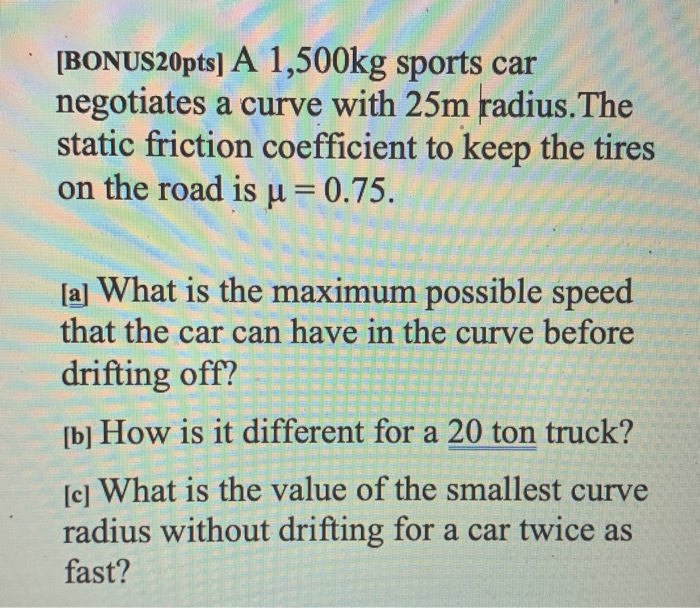[BONUS20pts] A 1,500kg sports car negotiates a curve with 25m radius.The static friction coefficient to keep the tires on the road is u = 0.75. [a] What is the maximum possible speed that the car can have in the curve before drifting off? [b] How is it different for a 20 ton truck? [c] What is the value of the smallest curve radius without drifting for a car twice as fast? CVP